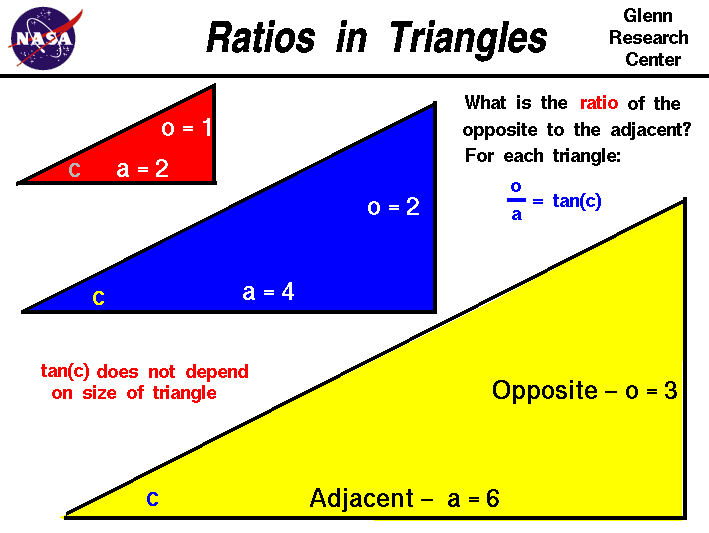+ Text Only Site
+ Non-Flash Version
+ Contact GlennTo better understand certain problems involving aircraft and propulsion it is necessary to use some mathematical ideas from trigonometry, the study of triangles. On another page we have introduced the trigonometric functions sine, cosine, and tangent of the angles of a right triangle and described how these functions relate the magnitude of the sides of a triangle. On this page we will further explore the relations between the sides of a right triangle and demonstrate that the trigonometric functions depend only the angles of the triangle and not on the size of the triangle. On this page we have constructed three right triangles of different size, but with the same acute angle c at the lower left. Using the terminology from the sine, cosine, and tangent page, we have made the side opposite the angle c equal to 1.0 for the red triangle. And we have made the side adjacent to angle c equal to 2.0. The ratio of the opposite to the adjacent for any right triangle is defined to be the tangent (tan) of the angle. For the red triangle the value of the tangent is: tan(c) = 1 / 2 = .5 For the blue triangle, we keep the angle c the same, but we have doubled the size of the opposite side and the adjacent side. Mathematicians would say that the blue and the red triangles are similar triangles; the angles are equal, but the size is different. What is the ratio of the opposite to the adjacent for the blue triangle? tan(c) = 2 / 4 = .5 The same value as for the red triangle. For the yellow triangle, we have tripled the size of the red triangle. The opposite side is now 3 units long and the adjacent side is now 6 units long. The yellow triangle is mathematically similar to the red and the blue triangle. What is the ratio of the opposite to the adjacent for the yellow triangle? tan(c) = 3 / 6 = .5 The same value as the red and the blue triangle. We could repeat this process for any multiple (m) of the sides of the red triangle and the result would be the same: tan(c) = (1 * m) / (2 * m) = .5 The value of the tangent (and the sine and cosine) depends on the size of the angle, not on the size of the triangle. The initial geometry of the red triangle was arbitrary. If we had picked other numbers for the ratio of the sides of the red triangle, the value of angle c would be different and the value of the tangent would be different. But the same value of the tangent would occur for the blue and yellow triangles as long as the angle c is kept the same. Activities: Guided Tours Navigation ..Beginner's Guide Home Page+ Inspector General Hotline + Equal Employment Opportunity Data Posted Pursuant to the No Fear Act + Budgets, Strategic Plans and Accountability Reports + Freedom of Information Act + The President's Management Agenda + NASA Privacy Statement, Disclaimer, and Accessibility CertificationEditor: Tom Benson NASA Official: Tom Benson Last Updated: Jun 12 2014 + Contact Glenn